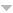Publications

Export 11 results:
Sort by: Author Title Type [ Year]
2021
Dimitrova, I., V. H. Fernandes, J. Koppitz, and T. M. Quinteiro. "Partial Automorphisms and Injective Partial Endomorphisms of a Finite Undirected Path." Semigroup Forum. 103 (2021): 87-105. AbstractWebsite

In this paper, we study partial automorphisms and, more generally, injective partial endomorphisms of a finite undirected path from Semigroup Theory perspective. Our main objective is to give formulas for the ranks of the monoids IEnd(P_n) and PAut(P_n) of all injective partial endomorphisms and of all partial automorphisms of the undirected path P_n with n vertices. We also describe Green's relations of PAut(P_n) and IEnd(P_n) and calculate their cardinals.

2020
Dimitrova, I., V. H. Fernandes, J. Koppitz, and T. M. Quinteiro. "Ranks of monoids of endomorphisms of a finite undirected path (DOI: 10.1007/s40840-019-00762-4)." Bulletin of the Malaysian Mathematical Sciences Society. 43 (2020): 1623-1645. AbstractWebsite

In this paper we study the widely considered endomorphisms and weak endomorphisms of a finite undirected path from monoid generators perspective. Our main aim is to determine the ranks of the monoids $wEnd P_n$ and $End P_n$ of all weak endomorphisms and all endomorphisms of the undirected path $P_n$ with $n$ vertices. We also consider strong and strong weak endomorphisms of $P_n$.

2017
Dimitrova, I., Vítor H. Fernandes, and J. Koppitz. "A note on generators of the endomorphism semigroup of an infinite countable chain." Journal of Algebra and its Applications (DOI: 10.1142/S0219498817500311). 16 (2017): 1750031 (9 pages). AbstractWebsite

In this note, we consider the semigroup $O(X)$ of all order endomorphisms of an infinite chain $X$ and the subset $J$ of $O(X)$ of all transformations $\alpha$ such that $|Im(\alpha)|=|X|$. For an infinite countable chain $X$, we give a necessary and sufficient condition on $X$ for $O(X) = < J >$ to hold. We also present a sufficient condition on $X$ for $O(X) = < J >$ to hold, for an arbitrary infinite chain $X$.

2013
Delgado, Manuel, and Vítor H. Fernandes. "Rees quotients of numerical semigroups." Portugaliae Mathematica. 70.2 (2013): 93-112. AbstractWebsite

We introduce a class of finite semigroups obtained by considering Rees
quotients of numerical semigroups.
Several natural questions concerning this class, as well as particular
subclasses obtained by considering some special ideals, are answered while
others remain open. We exhibit nice presentations for these semigroups and
prove that the Rees quotients by ideals of N, the positive integers under
addition, constitute a set of generators for the pseudovariety of commutative
and nilpotent semigroups.

2012
Dimitrova, I., Vítor H. Fernandes, and J. Koppitz. "The maximal subsemigroups of semigroups of transformations preserving or reversing the orientation on a finite chain." Publicationes Mathematicae Debrecen. 81.1-2 (2012): 11-29.
2006
Cordeiro, E., M. Delgado, and V. H. Fernandes. "Relative abelian kernels of some classes of transformation monoids." Bull. Austral. Math. Soc.. 73 (2006): 375-404.Website
2005
Delgado, Manuel, and Vítor H. Fernandes. "Solvable monoids with commuting idempotents." Int. J. Algebra Comput.. 15 (2005): 547-570. Abstract

The notion of the Abelian kernel of a finite monoid is a generalization of that of the derived subgroup of a finite group. A monoid $M$ is then called solvable if its chain of Abelian kernels terminates with the submonoid of $M$ generated by its idempotents. The main result of this paper is that the finite idempotent commuting monoids bearing this property are precisely those whose subgroups are solvable. In particular any finite aperiodic monoid is Abelian-solvable in this sense. A generalization of the main result of this paper has been published [in Int. J. Algebra Comput. 14, No. 5-6, 655-665 (2004; Zbl 1081.20067)] by the authors and ıt S. Margolis and ıt B. Steinberg.

2004
Delgado, Manuel, and Vítor H. Fernandes. "Abelian kernels of monoids of order-preserving maps and of some of its extensions." Semigroup Forum. 68 (2004): 335-356.Website
Delgado, Manuel, and Vítor H. Fernandes. "Abelian kernels, solvable monoids and the abelian kernel length of a finite monoid." Semigroups and languages. World Sci. Publ., River Edge, NJ, 2004. 68-85.
Delgado, Manuel, V{\'ı}tor H. Fernandes, Stuart Margolis, and Benjamin Steinberg. "On semigroups whose idempotent-generated subsemigroup is aperiodic." Internat. J. Algebra Comput.. 14 (2004): 655-665.Website
2000
Delgado, Manuel, and Vítor H. Fernandes. "Abelian kernels of some monoids of injective partial transformations and an application." Semigroup Forum. 61 (2000): 435-452.Website